# Motor Position PID Control

Publish Date: Nov 26, 2018 | 6 Ratings | 2.33 out of 5 | Print | Submit your review

## Overview

This tutorial shows the characteristics of the proportional (P), the integral (I), and the derivative (D) controls, and how to use them to obtain a desired response for the position of a DC motor. This tutorial uses LabVIEW and the LabVIEW Control Design and Simulation Module.

These tutorials are based on the Control Tutorials developed by Professor Dawn Tilbury of the Mechanical Engineering department at the University of Michigan and Professor Bill Messner of the Department of Mechanical Engineering at Carnegie Mellon University and were developed with their permission.

### 1. PID Design Method for DC Motor Position

From the main problem, the dynamic equation in transfer function form is the following: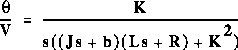The system schematic looks like: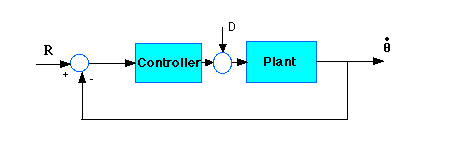For the original problem setup and the derivation of the above equations, please refer to the Modeling DC Motor Position page.

With a 1 rad/sec step reference, the design criteria are:

• Settling time less than 0.04 seconds
• Overshoot less than 16%
• No steady-state error due to a disturbance

### 2. Proportional Control

Now let's design a PID controller and add it into the system. Recall that the transfer function for a PID controller is: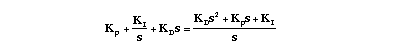Let's first try using a proportional controller with a gain of 1.7.

### LabVIEW Graphical Approach

Start by creating a VI to model the transfer function for the motor. For an explanation of how to create this model, refer to the Modeling DC Motor Position page.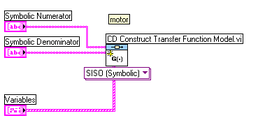Figure 1: Construct Motor Transfer Function

Next, create a transfer function for the controller. Add a second CD Construct Transfer Function Model VI from the Model Construction section of the Control Design palette. Create controls for the Symbolic Numerator and Variables inputs.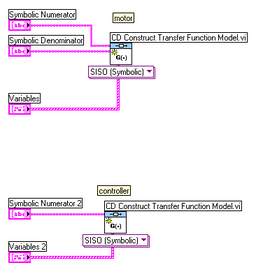Figure 2: Construct Controller Transfer Function

Put these models in series using a CD Series VI.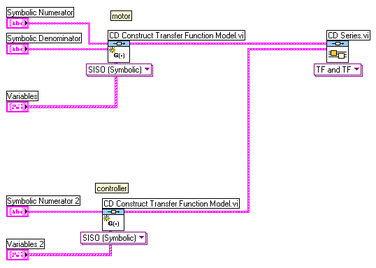Figure 3: Models in Series

Now add a CD Feedback VI, and connect the output from the CD Series VI to the Model 1 input of the CD Feedback VI. We use the Feedback VI to determine the closed-loop transfer function.

Next, create another transfer function model. Create a constant input for the numerator, and set this equal to 1. Connect this new transfer function to the Model 2 input of the CD Feedback VI.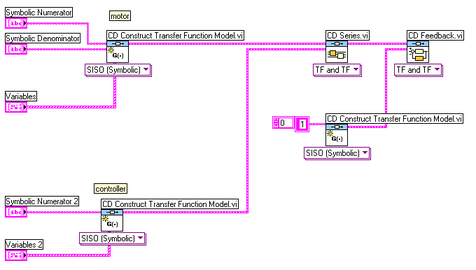Figure 4: Models in Series with Feedback VI

Now add a CD Step Response VI, connect the output from the CD Feedback VI to the input of the CD Step Response VI, and create a Time Response input and a Step Response Graph output for the CD Step Response VI. On the front panel, set the Time Info so that t0 = 0, dt = 0.001, and tf = 0.2.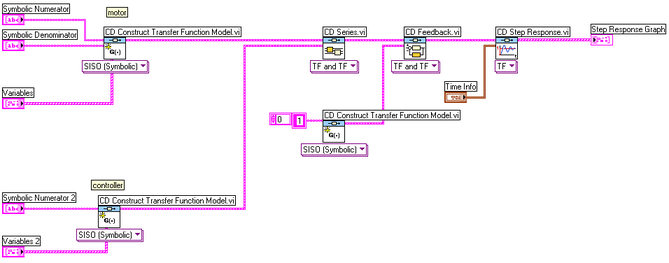Figure 5: Finished Block Diagram for Proportional Control

When you run the VI, you should see the following plot on the front panel: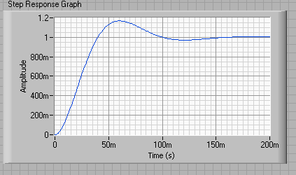Figure 6: Response to Step Input, Kp = 1.7

### Hybrid Graphical/MathScript Approach

Alternatively, you can achieve the same results using a hybrid graphical/MathScript approach. Begin by creating a blank VI, and add a MathScript Node. Paste the following code into your MathScript Node:

J=3.2284E-6;

b=3.5077E-6;

K=0.0274;

R=4;

L=2.75E-6;

num=K;

den=[(J*L) ((J*R)+(L*b)) ((b*R)+K^2) 0];

motor=tf(num,den);

Kp=1.7;

contr=Kp;

sys_cl=feedback(contr*motor,1);

We use the 'feedback' command to determine the closed-loop transfer function.

Create an output called sys_cl, and change its type to a TF Object. Add the CD Step Response VI to your block diagram, and connect the sys_cl output to the Transfer Function Model input of the Step Response VI. Finally, create a control for the Time Response terminal and an indicator for the Step Response Graph output. On the front panel, set t0 to 0, dt to .001, and tf to 0.2.

The block diagram should look like this: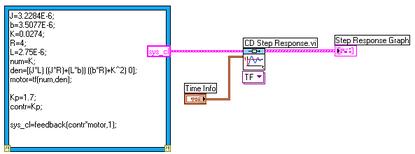Running this VI should result in a graph similar to the one in Figure 6.

### LabVIEW MathScript Approach

To achieve this result using MathScript, open the MathScript Window and paste the above code into the Command Window. Add the following lines of code to the end:

t=0:0.001:0.2;

step(sys_cl,t)

Running this code should result in a graph similar to Figure 6.

### 3. Step Disturbance Response

To look at the step disturbance response for the system, and if you are using a graphical approach, connect the motor and controller models to the inputs of another CD Feedback VI, then create inputs and outputs to the VI as we did before.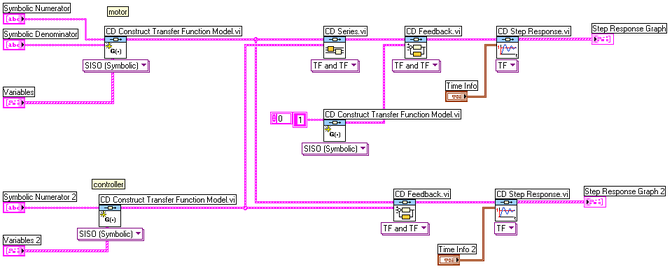If you are using a hybrid or MathScript approach, add the following code to your MathScript Node, and create an output for dist_cl:

dist_cl=feedback(motor,contr);

All of these methods should result in the following plot: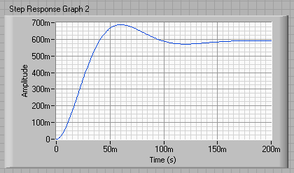Figure 9: Response to Step Disturbance, Kp = 1.7

### 4. PID Control

From the plots above, we see that although the steady-state error looks good, the settling time is too large, as is the overshoot. We also see that the steady-state error to a disturbance is large.

Recall from the PID tutorial page that adding an integral term will eliminate the steady-state error and a derivative term will reduce the overshoot. Let's first try a PI controller to get rid of the disturbance steady state error.

### LabVIEW Graphical Approach

To achieve this with a graphical approach, add on to the VI that we created earlier. Create a control for the Symbolic Denominator input for the controller transfer function VI.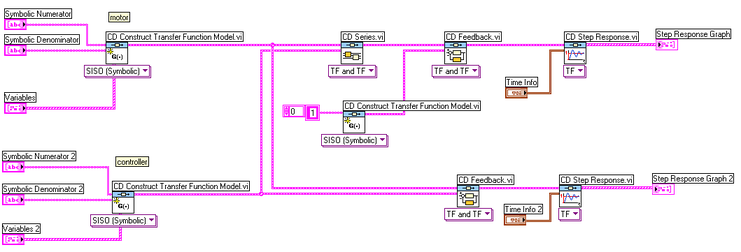Change your front panel values so that the values for the controller numerator are "Ki   Kp" and the values for its denominator are "0  1". Change the controller variables on the front panel so that the variable Kp has a value of 1.7, and Ki has a value of 20.

Running this VI should give you the following Step Response plot: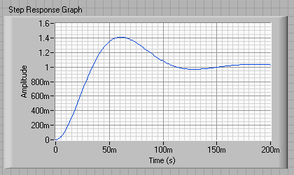Figure 11: Response to Step Input, Kp = 1.7, Ki = 20

Also, you should see the following Step Disturbance plot: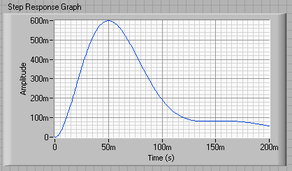Figure 12: Response to Step Disturbance, Kp = 1.7, Ki = 20

The integral control has reduced the steady state error to zero.

### Hybrid Graphical/MathScript Approach

If you are using a hybrid approach, change your MathScript code so that it looks like the following:

J=3.2284E-6;

b=3.5077E-6;

K=0.0274;

R=4;

L=2.75E-6;

num=K;

den=[(J*L) ((J*R)+(L*b)) ((b*R)+K^2) 0];

motor=tf(num,den);

Kp=1.7;

Ki=20;

contr=tf([Kp Ki],[1 0]);

sys_cl=feedback(contr*motor,1);

Running this VI should result in a similar response to Figure 11.

To see what happened to the step disturbance response, add the following code to your MathScript Node:

dist_cl=feedback(motor,contr);

Change the sys_cl output name to dist_cl and run the VI. You should see a plot similar to Figure 12.

### 5. Tuning the Gains

Although the steady state error has been removed, the settling time is still too long. Let's increase the gains in order to speed up the response. Change Ki to 200 and Kp to 17. Rerun your VI, and you should get plots like these: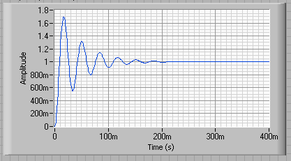Figure 13: Response to Step Input, Kp = 17, Ki = 200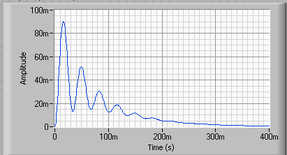Figure 14: Response to Step Disturbance, Kp = 17, Ki = 200

Now we see that the response is faster than before, but the large Ki has worsened the transient response (big overshoot). Let's now try adding a derivative term to reduce the overshoot.

If you are using a graphical approach, change your front panel values so that the controller numerator has "Ki  Kp  Kd", the denominator has "0  1", and the variables have Kp = 17, Ki = 200, and Kd = 0.15.

To do this with the hybrid approach, make the following changes to your MathScript code:

Kp=17;

Ki=200;

Kd=0.15;

contr=tf([Kd Kp Ki],[1 0]);

sys_cl=feedback(contr*motor,1);

You should now get this plot: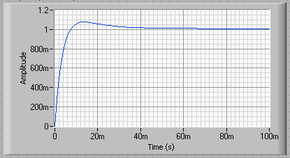Figure 15: Response to Step Input, Kp = 17, Ki = 200, Kd = 0.15

And your step disturbance plot should look like this: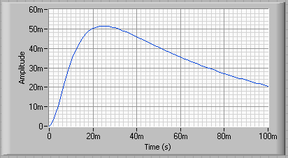Figure 16: Response to Step Disturbance, Kp = 17, Ki = 200, Kd = 0.15

We now see that our step response looks really good. It has less than 16% overshoot, the settling time is roughly 40 ms, and there is no steady-state error.

However, the step disturbance response is now really slow. Lets increase Ki to speed up the disturbance response. Change Ki to 600 and rerun your VI. You should get the following plots: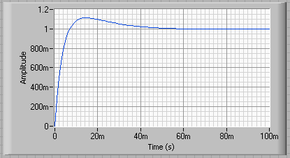Figure 17: Response to Step Input, Kp = 17, Ki = 600, Kd = 0.15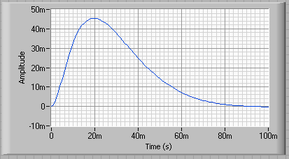Figure 18: Response to Step Disturbance, Kp = 17, Ki = 600, Kd = 0.15

We now can see that the step response has a settling time of roughly 40 ms, it has less than 16% overshoot, and it has no steady state error. The step disturbance response also has no steady state error. So now we know that if we use a PID controller with

Kp=17
Ki=600
Kd=.15

all of our design requirements will be satisfied.